Home

# CATEGORICAL PROOF OF HOLOMORPHIC ATIYAH–BOTT FORMULA

## Abstract

Given a$2$ -commutative diagram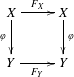in a symmetric monoidal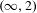$(\infty ,2)$ -category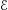$\mathscr{E}$ where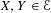$X,Y\in \mathscr{E}$ are dualizable objects and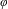$\unicode[STIX]{x1D711}$ admits a right adjoint we construct a natural morphism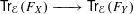$\mathsf{Tr}_{\mathscr{E}}(F_{X})\xrightarrow[{}]{~~~~~}\mathsf{Tr}_{\mathscr{E}}(F_{Y})$ between the traces of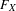$F_{X}$ and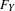$F_{Y}$ , respectively. We then apply this formalism to the case when$\mathscr{E}$ is the$(\infty ,2)$ -category of$k$ -linear presentable categories which in combination of various calculations in the setting of derived algebraic geometry gives a categorical proof of the classical Atiyah–Bott formula (also known as the Holomorphic Lefschetz fixed point formula).

## References

Hide All
MathJax
MathJax is a JavaScript display engine for mathematics. For more information see http://www.mathjax.org.

# CATEGORICAL PROOF OF HOLOMORPHIC ATIYAH–BOTT FORMULA

## Metrics

### Full text viewsFull text views reflects the number of PDF downloads, PDFs sent to Google Drive, Dropbox and Kindle and HTML full text views.

Total number of HTML views: 0
Total number of PDF views: 0 *Loading metrics...

### Abstract viewsAbstract views reflect the number of visits to the article landing page.

Total abstract views: 0 *Loading metrics...

* Views captured on Cambridge Core between <date>. This data will be updated every 24 hours.

Usage data cannot currently be displayed.